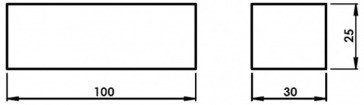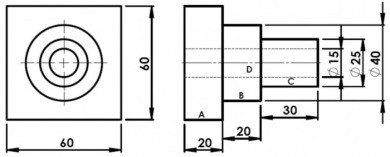Saturday, December 9, 2023

# Weight Calculation

Simple examples for calculating weight are given here.

1) Calculate the weight of the cast iron rectangular bar as shown in fig. Assume density of cast iron as 0.0072 gm/mm^3.Solution:

Volume = length x breadth x height
= 100 x 30 x 25
= 75000 mm^3
Hence,
Weight of the rectangular bar= Volume x density of Cast iron
= 75000 x 0.0072
= 540 gm
= 0.54 kg.

2) Calculate the weight of the mild steel part as shown in fig. Assume density of mild steel as 0.0078 gm/mm^3.Solution:
Break up the part into four following simple parts as A, B, C & D.
Volume of A = l x b x h
= 60 x 20 x 60
= 72000 mm^3
Volume of B = (π x d^2 x l)/4
= (π x 40^2 x 20)/4
= 8000 π mm^3

Volume of C = (π x d^2 x l)/4
= (π x 25^2 x 30)/4
= 4687.5 π mm^3

Volume of D = (π x d^2 x l)/4
= (π x 15^2 x 70)/4
= 3937.5 π mm^3

Then,

Total Volume = Volume of A + Volume of B + Volume of C – Volume of D
= 72000 + 8000 π + 4687.5 π – 3937.5 π
= 72000 + (8750 π)
= 99492.5 mm^3
Hence,
Weight of the part = Total volume x density of mild steel
= 99492.5 x 0.0078
= 776.0415 gm
= 0.776 kg.

Orthographic Projection